Related Articles
Python | Working with Pandas and XlsxWriter | Set – 1
• Difficulty Level : Medium
• Last Updated : 26 Dec, 2018

Python Pandas is a data analysis library. It can read, filter and re-arrange small and large datasets and output them in a range of formats including Excel.

Pandas writes Excel files using the XlsxWriter modules.

`XlsxWriter` is a Python module for writing files in the `XLSX `file format. It can be used to write text, numbers, and formulas to multiple worksheets. Also, it supports features such as formatting, images, charts, page setup, auto filters, conditional formatting and many others.

Code #1: Converting a Pandas dataframe to an xlsx file using Pandas and XlsxWriter.

 `# import pandas as pd``import` `pandas as pd`` ` `# Create a Pandas dataframe from some data.``df ``=` `pd.DataFrame({``'Data'``: [``'Geeks'``, ``'For'``, ``'geeks'``, ``'is'``,``                               ``'portal'``, ``'for'``, ``'geeks'``]})`` ` `# Create a Pandas Excel writer``# object using XlsxWriter as the engine.``writer ``=` `pd.ExcelWriter(``'pandasEx.xlsx'``, ``                   ``engine ``=``'xlsxwriter'``)`` ` `# Write a dataframe to the worksheet.``df.to_excel(writer, sheet_name ``=``'Sheet1'``)`` ` `# Close the Pandas Excel writer``# object and output the Excel file.``writer.save()`

Output :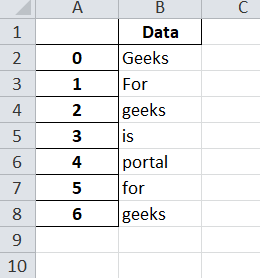Code #2: Writing multiple dataframes to worksheets using Pandas and XlsxWriter.

 `# import pandas as pd``import` `pandas as pd`` ` ` ` `# Create some Pandas dataframes from some data.``df1 ``=` `pd.DataFrame({``'Data'``: [``11``, ``12``, ``13``, ``14``]})``df2 ``=` `pd.DataFrame({``'Data'``: [``21``, ``22``, ``23``, ``24``]})``df3 ``=` `pd.DataFrame({``'Data'``: [``31``, ``32``, ``33``, ``34``]})`` ` `# Create a Pandas Excel writer object ``# using XlsxWriter as the engine.``writer ``=` `pd.ExcelWriter(``'pandas_multiple.xlsx'``, ``                          ``engine ``=``'xlsxwriter'``)`` ` `# Write each dataframe to a different worksheet.``df1.to_excel(writer, sheet_name ``=``'Sheet1'``)``df2.to_excel(writer, sheet_name ``=``'Sheet2'``)``df3.to_excel(writer, sheet_name ``=``'Sheet3'``)`` ` `# Close the Pandas Excel writer object``# and output the Excel file.``writer.save()`

Output :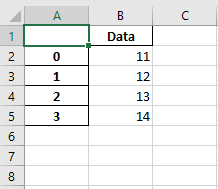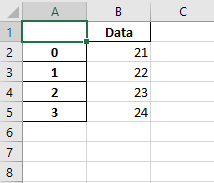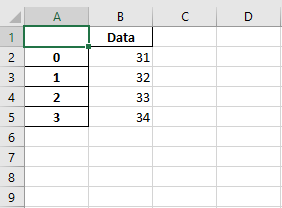Code #3: Positioning dataframes in a worksheet using Pandas and XlsxWriter.

 `# import pandas as pd``import` `pandas as pd`` ` ` ` `# Create some Pandas dataframes from some data.``df1 ``=` `pd.DataFrame({``'Data'``: [``11``, ``12``, ``13``, ``14``]})``df2 ``=` `pd.DataFrame({``'Data'``: [``21``, ``22``, ``23``, ``24``]})``df3 ``=` `pd.DataFrame({``'Data'``: [``31``, ``32``, ``33``, ``34``]})``df4 ``=` `pd.DataFrame({``'Data'``: [``41``, ``42``, ``43``, ``44``]})`` ` `# Create a Pandas Excel writer object``# using XlsxWriter as the engine.``writer ``=` `pd.ExcelWriter(``'pandas_positioning.xlsx'``, ``                             ``engine ``=``'xlsxwriter'``)`` ` `# write and Positioning the dataframes in the worksheet.``# Default position, cell A1.``df1.to_excel(writer, sheet_name ``=``'Sheet1'``)  ``df2.to_excel(writer, sheet_name ``=``'Sheet1'``, startcol ``=` `3``)``df3.to_excel(writer, sheet_name ``=``'Sheet1'``, startrow ``=` `6``)`` ` `# It is also possible to write the``# dataframe without the header and index.``df4.to_excel(writer, sheet_name ``=``'Sheet1'``,``             ``startrow ``=` `7``, startcol ``=` `4``,``             ``header ``=` `False``, index ``=` `False``)`` ` `# Close the Pandas Excel writer object``# and output the Excel file.``writer.save()`

Output :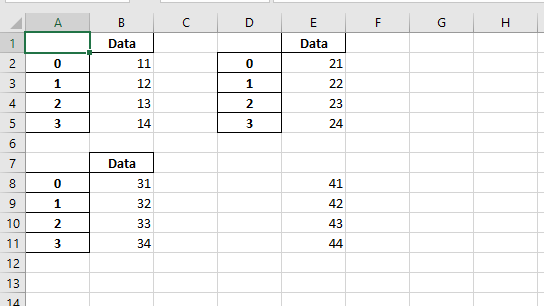Attention geek! Strengthen your foundations with the Python Programming Foundation Course and learn the basics.

To begin with, your interview preparations Enhance your Data Structures concepts with the Python DS Course.

My Personal Notes arrow_drop_up